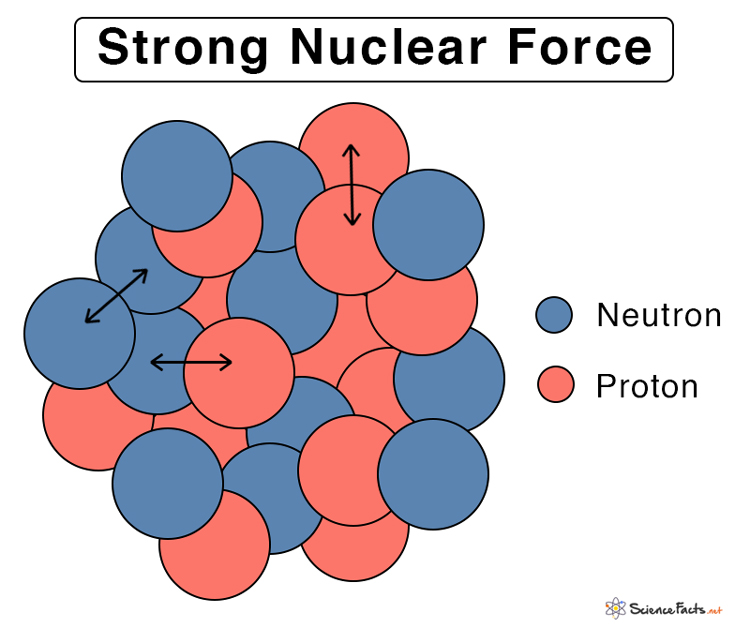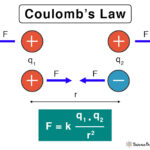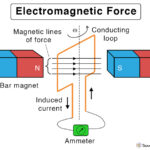Home / Physics / Strong Nuclear Force

# Strong Nuclear Force

## What is Strong Nuclear Force

The strong nuclear force is a force between the fundamental particles that constitute the nucleus. It can be between two protons, two neutrons, or a proton and a neutron. The interaction between quarks, which are the smallest particles that constitute protons and neutrons, is also mediated through the strong force. It is an attractive force responsible for holding the particles together, thereby making atoms and matter stable. The strong interaction is the mechanism responsible for the strong nuclear force.Strong Nuclear Force

History and Discovery of the Strong Nuclear Force

The first theory of the strong nuclear force was discovered in 1935 by Japanese physicist Hideki Yukawa, who discovered the force-carrying particles known as mesons. He suggested that nucleons exchanging particles create a force field.

## Strength and Range of Strong Nuclear Force

The strong nuclear force is one of the four fundamental forces of nature, including weak nuclear force, gravitational force, and electromagnetic force. It is 137 times stronger than the electromagnetic force, about a million times stronger than the weak nuclear force, and 1.67 x 1038 times stronger than the gravitational force. It has a short and finite range, which means that the particles have to be somewhere within 10-15 meters from each other or roughly within a proton’s diameter.

Relative Strength and Range of Fundamental Forces

## How does the Strong Nuclear Force Work

Two positively charged protons repel each other because of the electromagnetic force. Therefore, the strong nuclear force overcomes the powerful repulsion between protons in the atomic nucleus. The stronger the force, the higher is energy transferred during the interaction. Hence, a large amount of energy is stored in the nucleus. When the force between the protons and neutrons is broken, extreme high-energy photons are released.

The particles responsible for carrying the strong nuclear force are known as gluons. It is so-called because the particles are like ‘glue,’ that holds the protons, neutrons, and quarks together. An exchange of gluons causes the strong nuclear force.

The theory that describes the strong force is called quantum chromodynamics (QCD).

## Properties of Strong Nuclear Force

Here are some facts and characteristics of the strong nuclear force.

• The most potent force in nature
• Exchange force
• Short-range (~10-15 m)
• Non-central and non-conservative
• Spin-dependent and charge-independent
• Does not follow product law and the inverse-square law
• 137 times stronger than the electromagnetic force, 106 times stronger than the weak nuclear force, and 1.67 x 1038 times stronger than the gravitational force.

## Examples of Strong Nuclear Force

The only example of the strong nuclear force is the force between the fundamental particles that constitute the nucleus. Matter is made up of atoms, which are in turn made up of nuclei and electrons. Everyday objects like mobile phones, computers, chairs, and tables exist because of the strong force. This force powers the Sun and other stars. It supplies energy to a nuclear reactor in nuclear power plants.

Article was last reviewed on Monday, April 5, 2021

### Related articlesCoulomb’s LawElectromagnetic ForceNuclear Force